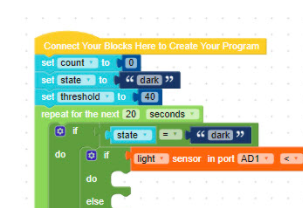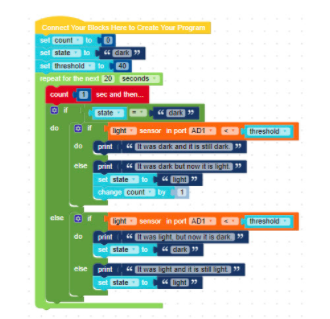## 07 Mission Instructions

Your mission is to create a robot that counts how many times the lights are turned off and on each day for later analysis.

### 6. Code: State MachineThen, we can use an [if-do-else] block to answer the first part of our question (was the light on or off before we checked the sensor). If the state is set to “dark” then…. (And we know that it *is* because we told the robot it was dark when we created the variable above!).

Now the robot will need to figure out whether it is acting based on #3 or #4 from the list of states above. That means the choices are:

3. The light was previously off and it stays off .

4. The light was previously off, but it has been turned on.

So we need to add an if-do-else block inside our first if-do-else block. If the light sensor reading is less than the threshold reading, then the lights stayed off. Let’s have our program print the sentence in number 3 above so we know exactly what is happening when we test our robot.

Otherwise (Else), print “the light used to be off, but it has been turned on.” AND we’ll need to change the state variable to “light” (because the lights are on now!).Now let’s copy that if-do-else block and paste it in the Else section of the larger if-do-else block. Time to change the sentences to match #1 and #2 above!

1. The light was previously on and stays on.

2. The light was previously on, but it was turned off .

Take a minute to read the program out loud and check that your print-outs make sense. Start from the repeat block and read down one at a time.

“If the state is dark, then the sensor reading is less than the threshold?” That means it was dark and it is still dark.

“If the state is dark, then the sensor reading is not less than the threshold.” That means it was dark and it is now light.

“If the state was not dark, then the sensor reading is less than the threshold.” That means it was light and it is now dark.

We’re building a state machine that should count every time the lights in the room change state, right? So that means we need to add in commands when we want the count to change. See if you can figure out where to put the change [count] by 1 block.

Now that we have a program that counts how many times the lights are turned on and off, we need to make sure it reports the number back to us at the end. How could you do that with a “print” command?